# Boundary Traversal of a Binary Tree

Problem Statement: BoundaryTraversal of a binary tree. Write a program for the Anti-Clockwise Boundary traversal of a binary tree.

```Example: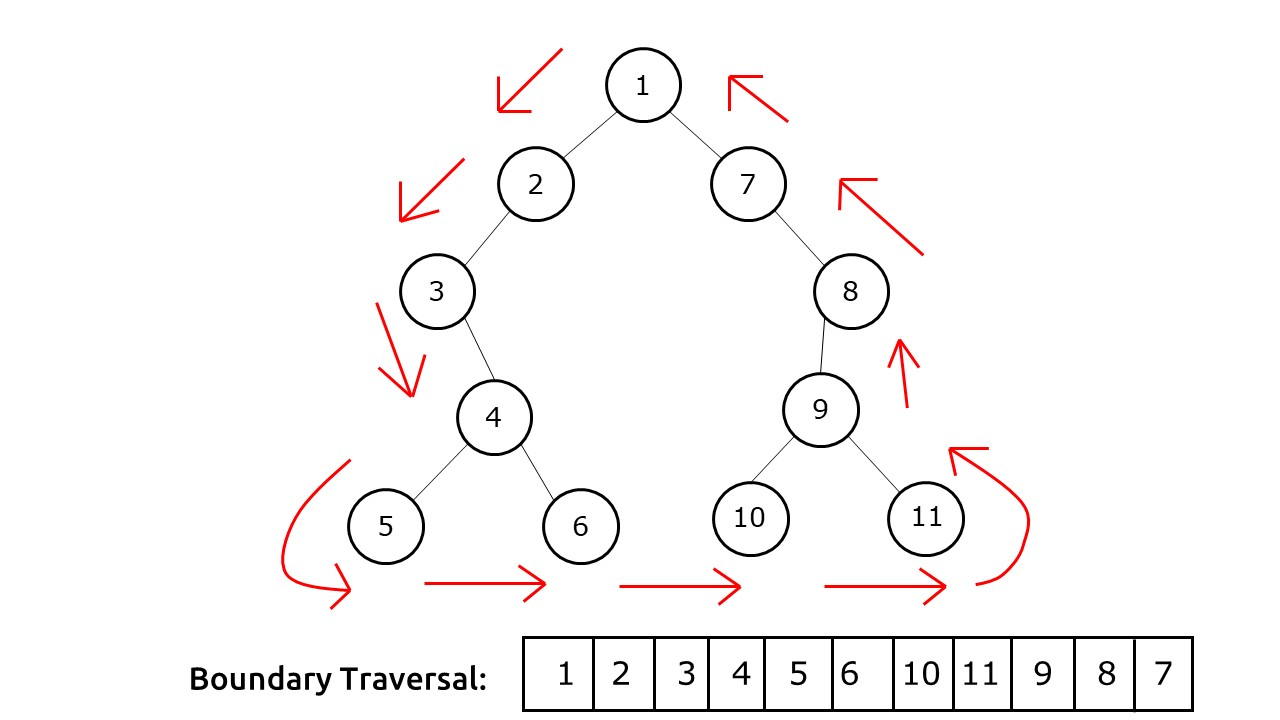```

## Solution :

Disclaimer: Don’t jump directly to the solution, try it out yourself first.

Approach: Boundary traversal in an anti-clockwise direction can be described as a traversal consisting of three parts:

1. Part 1: Left Boundary of the tree (excluding the leaf nodes).
2. Part 2: All the leaf nodes travelled in the left to right direction.
3. Part 3: Right Boundary of the tree (excluding the leaf nodes), traversed in the reverse direction.

We take a simple data structure like a vector/Arraylist to store the Boundary Traversal. The root node is coming from both the boundaries (left and right). Therefore, to avoid any confusion, we push it on our list at the very start.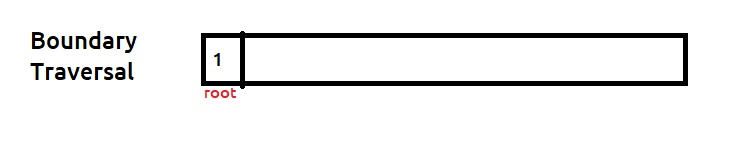We will now see the approach to finding these three parts.

Part 1:  Left Boundary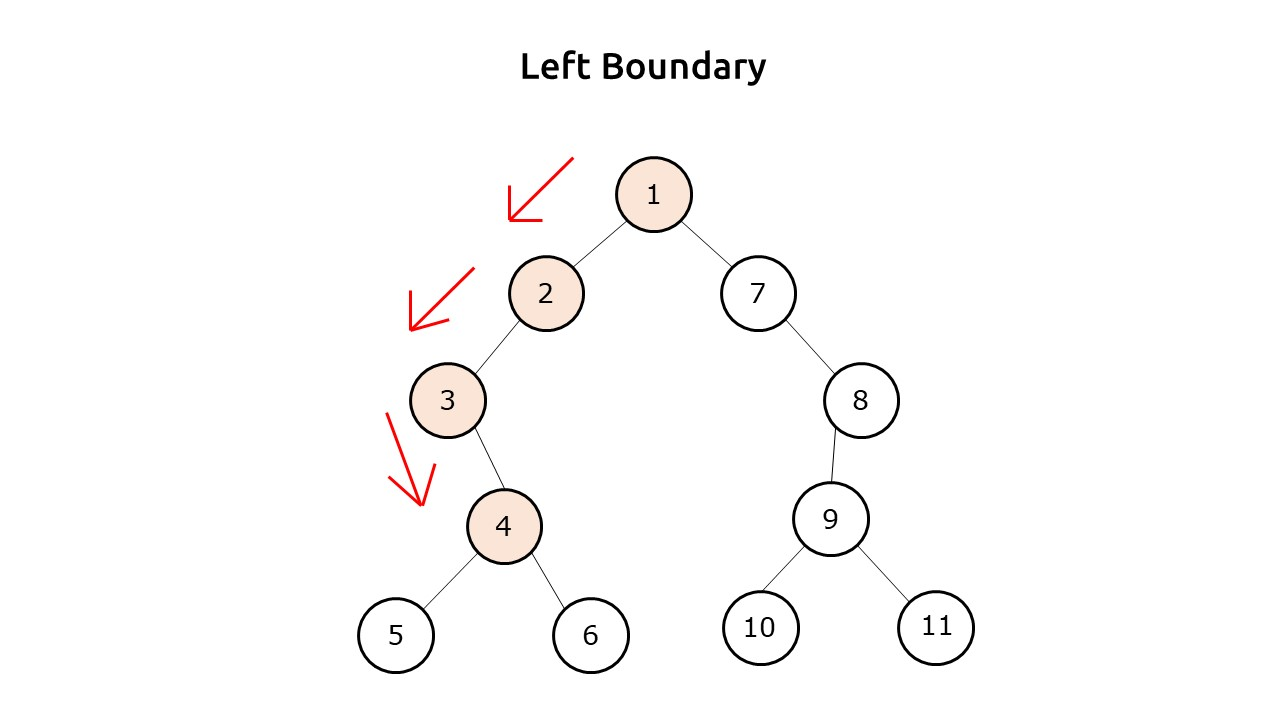To traverse the left boundary, we can set a simple iteration. Initially, we make the cur pointer point to the root’s left. In every iteration, if the cur node is not a leaf node, we print it. Then we always try to move left of the cur pointer. If there is no left child, then we move to the right of cur and in the next iteration, again try to move to the left first. We stop our execution when cur is pointing to NULL.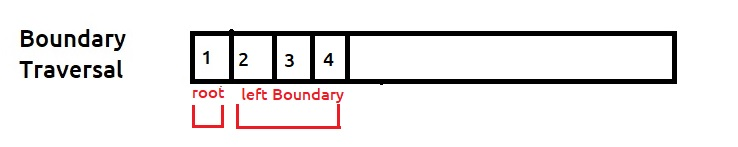Part 2: Leaf nodes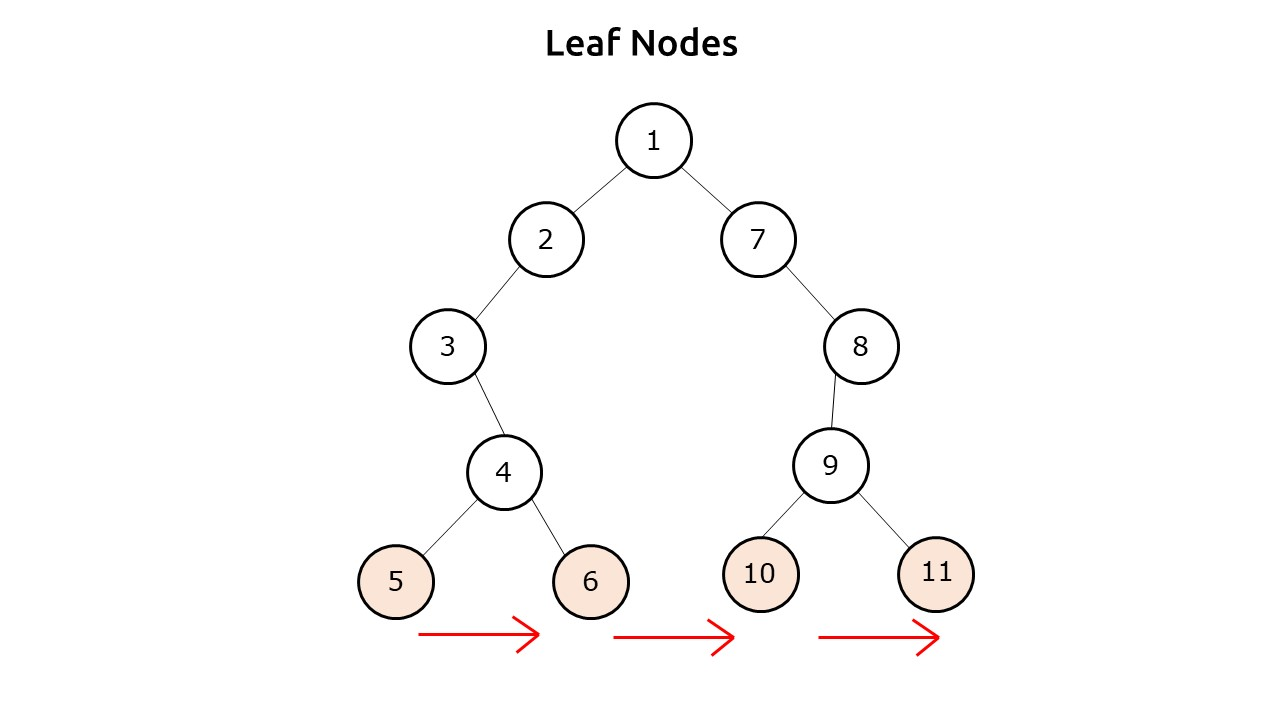To print the leaf nodes, we can do a simple preorder traversal, and check if the current node is a leaf node or not. If it is a leaf node just print it.

Please note that we want the leaves to come in a specific order which is bottom-left to top-right, therefore a level order traversal will not work because it will print the upper-level leaves first. Therefore, we use a preorder traversal.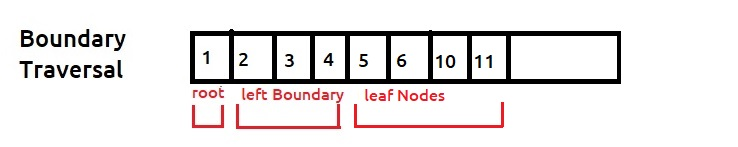Part 3: Right Boundary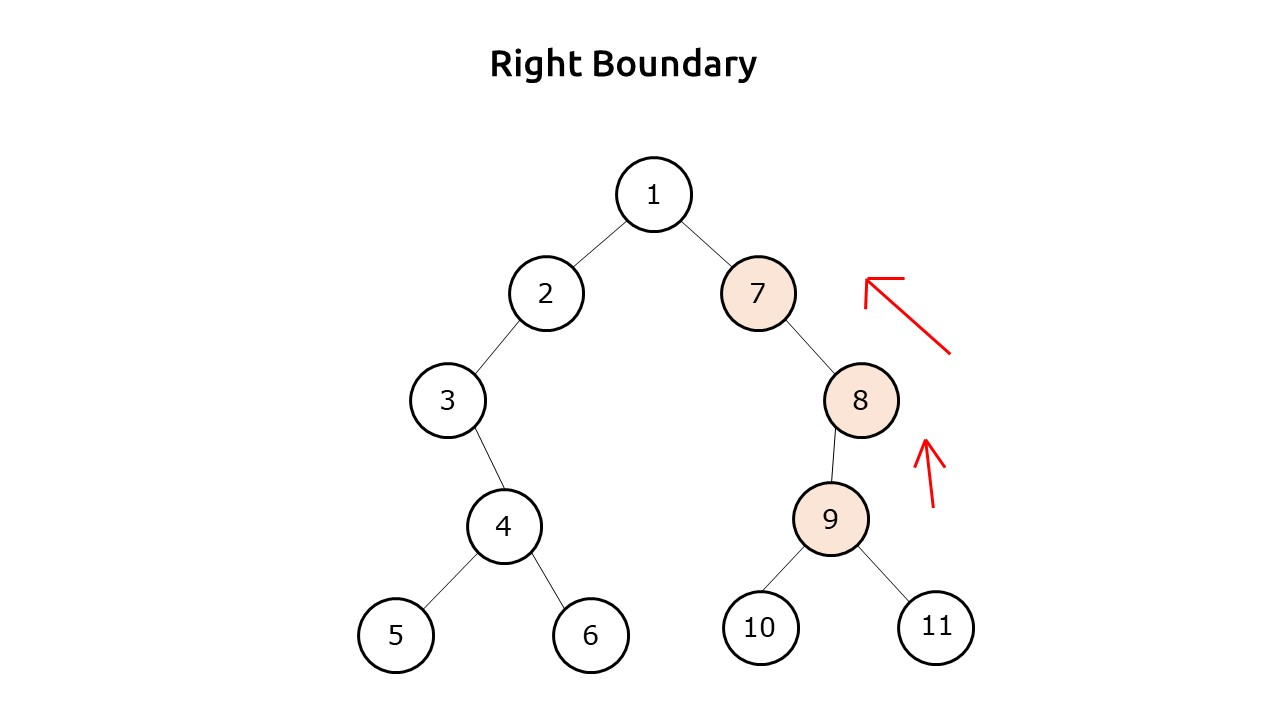We need to print the right boundary in the Reverse direction. It is very similar to the left boundary traversal. We initialize our cur pointer to the right child of the root node and set an iterative loop. To achieve the reverse direction, we take an auxiliary list. In every iteration, we check if the current node is not a leaf node then we push it to the auxiliary list. Then we first try to move right of cur, if there is no right child only then we move left. We stop our execution once cur points to NULL.

Now the auxiliary list contains the nodes of the right boundary. We iterate from the end to start off this list and in every iteration, push the value to our main boundary traversal list. This way we get the nodes in the reverse direction.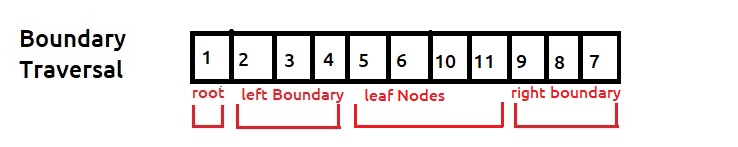Dry Run: In case you want to watch the dry run for this approach, please watch the video attached below.

Code:

## C++ Code

``````#include <bits/stdc++.h>

using namespace std;

struct node {
int data;
struct node * left, * right;
};

bool isLeaf(node * root) {
return !root -> left && !root -> right;
}

void addLeftBoundary(node * root, vector < int > & res) {
node * cur = root -> left;
while (cur) {
if (!isLeaf(cur)) res.push_back(cur -> data);
if (cur -> left) cur = cur -> left;
else cur = cur -> right;
}
}
void addRightBoundary(node * root, vector < int > & res) {
node * cur = root -> right;
vector < int > tmp;
while (cur) {
if (!isLeaf(cur)) tmp.push_back(cur -> data);
if (cur -> right) cur = cur -> right;
else cur = cur -> left;
}
for (int i = tmp.size() - 1; i >= 0; --i) {
res.push_back(tmp[i]);
}
}

void addLeaves(node * root, vector < int > & res) {
if (isLeaf(root)) {
res.push_back(root -> data);
return;
}
if (root -> left) addLeaves(root -> left, res);
if (root -> right) addLeaves(root -> right, res);
}

vector < int > printBoundary(node * root) {
vector < int > res;
if (!root) return res;

if (!isLeaf(root)) res.push_back(root -> data);

return res;
}

struct node * newNode(int data) {
struct node * node = (struct node * ) malloc(sizeof(struct node));
node -> data = data;
node -> left = NULL;
node -> right = NULL;

return (node);
}

int main() {

struct node * root = newNode(1);
root -> left = newNode(2);
root -> left -> left = newNode(3);
root -> left -> left -> right = newNode(4);
root -> left -> left -> right -> left = newNode(5);
root -> left -> left -> right -> right = newNode(6);
root -> right = newNode(7);
root -> right -> right = newNode(8);
root -> right -> right -> left = newNode(9);
root -> right -> right -> left -> left = newNode(10);
root -> right -> right -> left -> right = newNode(11);

vector < int > boundaryTraversal;
boundaryTraversal = printBoundary(root);

cout << "The Boundary Traversal is : ";
for (int i = 0; i < boundaryTraversal.size(); i++) {
cout << boundaryTraversal[i] << " ";
}
return 0;
}``````

Output:

The Boundary Traversal is : 1 2 3 4 5 6 10 11 9 8 7

Time Complexity: O(N).

Reason: The time complexity will be O(H) + O(H) + O(N) which is ≈ O(N)

Space Complexity: O(N)

Reason: Space is needed for the recursion stack while adding leaves. In the worst case (skewed tree), space complexity can be O(N).

## Java Code

``````import java.util.*;
class Node {
int data;
Node left, right;
Node(int data) {
this.data = data;
left = null;
right = null;
}
}
public class Main {
static Boolean isLeaf(Node root) {
return (root.left == null) && (root.right == null);
}

static void addLeftBoundary(Node root, ArrayList < Integer > res) {
Node cur = root.left;
while (cur != null) {
if (cur.left != null) cur = cur.left;
else cur = cur.right;
}
}
static void addRightBoundary(Node root, ArrayList < Integer > res) {
Node cur = root.right;
ArrayList < Integer > tmp = new ArrayList < Integer > ();
while (cur != null) {
if (cur.right != null) cur = cur.right;
else cur = cur.left;
}
int i;
for (i = tmp.size() - 1; i >= 0; --i) {
}
}

static void addLeaves(Node root, ArrayList < Integer > res) {
if (isLeaf(root)) {
return;
}
if (root.left != null) addLeaves(root.left, res);
if (root.right != null) addLeaves(root.right, res);
}
static ArrayList < Integer > printBoundary(Node node) {
ArrayList < Integer > ans = new ArrayList < Integer > ();
return ans;
}

public static void main(String args[]) {

Node root = new Node(1);
root.left = new Node(2);
root.left.left = new Node(3);
root.left.left.right = new Node(4);
root.left.left.right.left = new Node(5);
root.left.left.right.right = new Node(6);
root.right = new Node(7);
root.right.right = new Node(8);
root.right.right.left = new Node(9);
root.right.right.left.left = new Node(10);
root.right.right.left.right = new Node(11);

ArrayList < Integer > boundaryTraversal;
boundaryTraversal = printBoundary(root);

System.out.println("The Boundary Traversal is : ");
for (int i = 0; i < boundaryTraversal.size(); i++) {
System.out.print(boundaryTraversal.get(i) + " ");
}

}
}``````

Output:

The Boundary Traversal is : 1 2 3 4 5 6 10 11 9 8 7

Time Complexity: O(N).

Reason: The time complexity will be O(H) + O(H) + O(N) which is ≈ O(N)

Space Complexity: O(N)

Reason: Space is needed for the recursion stack while adding leaves. In the worst case (skewed tree), space complexity can be O(N).